Lo how a rose e'er blooming
From tender stem hath sprung.

As I write this blog post, a radio station is playing Chrismas music. One of my favorite Christmas songs is the old German hymn that many of us know as "Lo, How a Rose E're Blooming." I was humming the song recently when someone asked me how to use SAS to draw curves in polar coordinates. I immediately thought about "polar roses," mathematical parametric curves of the form r = cos(k θ).

In the spirit of Christmas, I present "Lo, how a polar rose e'er blooming, from SGPLOT hath sprung."

### Plotting polar coordinates in SAS

It is easy to graph a polar curve in SAS. Here's a quick overview, or a "polar express" tour, if you prefer.

Many familiar polar equations are parametric curves. The simplest are relations are of the form r = f(θ), where θ is the polar angle. The following SAS DATA step creates evenly spaced values of theta in the range [0, 2π]. For each value of theta, it computes r = cos(k θ), which is the equation for a rose with k petals. The polar coordinates (r, θ) are converted to Euclidean coordinates through the usual transformation x = r*cos(theta) and y = r*sin(theta).

After creating the points along the curve, you can visualize it by using PROC SGPLOT. The SERIES statement is used to display the parametric curve in Euclidean coordinates. The XAXIS and YAXIS statements use the MIN= and MAX= options to ensure that the image appears in a square region, and the ASPECT=1 option is used to ensure that the aspect ratio of the plot does not distort the geometry of the rose.

```%let k = 5; /* draw the curve r=cos(k * theta) in polar coords, which is a k-leaved rose */ data Rose; do theta = 0 to 2*constant("pi") by 0.01; r = cos(&k * theta); /* rose */ x = r*cos(theta); /* convert to Euclidean coords */ y = r*sin(theta); output; end; run;   title "Polar Rose: r = cos(&k theta)";; proc sgplot data=Rose aspect=1; refline 0 / axis=x; refline 0 / axis=y; series x=x y=y; xaxis min=-1 max=1; yaxis min=-1 max=1; run;```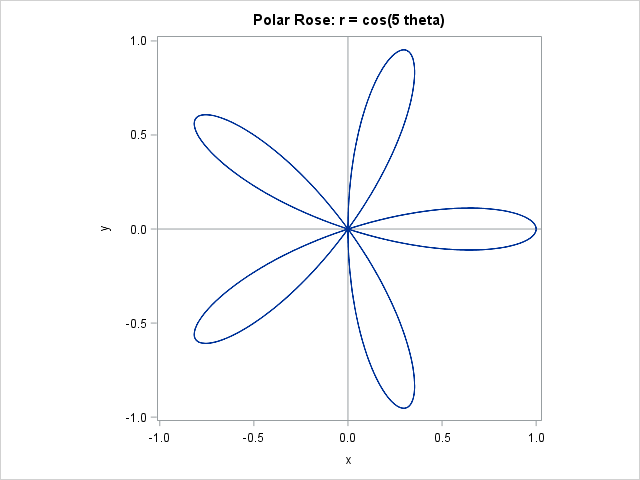### Graphing generalized roses

The Wikipedia page for the polar rose contains a brief discussion about what the curve r = cos(k θ) looks like when k is a rational number n/d. When k is not an integer, most of the curves do not look like a rose—or any other kind of flower! Only a myopic mathematician would call some of these images roses.

Regardless, let's see how to get some of these "roses" to bloom in SAS. The following DATA step generates all positive rational numbers of the form n/d, where n ≤ 7 and d ≤ 9 are integers. For each value of k, the program computes the generalize rose equation and converts the values from polar to Euclidean coordinates:

```data Roses; do n = 1 to 7; do d = 1 to 9; k = n / d; /* draw the rose r=cos(n/d * theta) */ do theta = 0 to 2*lcm(n,d)*constant("pi") by 0.05; r = cos(k * theta); /* generalized rose */ x = r*cos(theta); /* convert to Euclidean coords */ y = r*sin(theta); output; end; end; end; run;```

It is not any harder to visualize 63 roses than it is to visualize one. The SGPANEL procedures was designed to display multiple cells, each with the same type of graph. Therefore, to display an entire bouquet of roses, you merely have to specify the N and D variables on the PANELBY statement, and SAS handles the rest:

```title "Polar Roses: r = cos(n/d theta)"; proc sgpanel data=Roses aspect=1; panelby n d / layout=lattice onepanel rowheaderpos=left; refline 0 / axis=x transparency=0.5; refline 0 / axis=y transparency=0.5; series x=x y=y; colaxis display=none; rowaxis display=none; run;```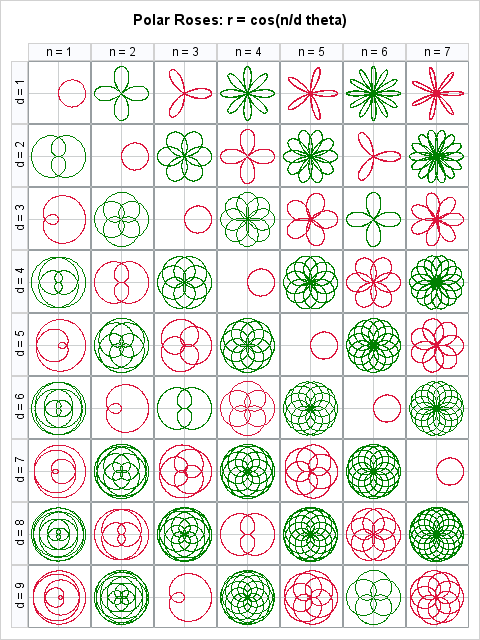You can see certain numeric patterns in the lattice display. In particular, when n/d is a reducible fraction (such as 2/4, 3/6, and 4/8), the image is identical to the reduced fraction (such as 1/2). Do you see other patterns? Leave a comment.

These roses are beautiful, like the song that inspired me to create them. Since I get up early in the morning to write my blog, a few additional lyrics seem appropriate:

It came a blossom bright.
Amid the cold of winter
When half spent was the night.

Happy holidays to all my readers. I am taking a short vacation and will return in 2016. Until then, keep your eyes open: math, statistics, and beauty are all around you, ever blooming!

tags: Just for Fun, Math

The post Lo, how a polar rose e'er blooming appeared first on The DO Loop.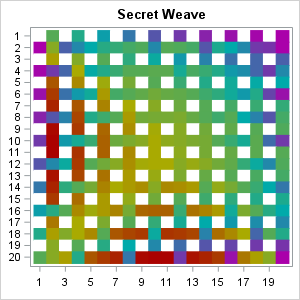In my previous blog post, I showed how you can use SAS to program a "weaving" algorithm that takes an image, cuts it into strips, and weaves the strips together to create mathematical art. I used matrices and heat maps for the computations and visualization.

At the end of the post, I presented an image of woven strips (shown at left) and challenged the reader to "deduce the original image from the weave." This article shows that you can "unweave" the image, reassemble the strips, and use interpolation to obtain an approximation to the original image. You can download the SAS/IML program that computes and visualizes the preimage.

### Unweaving a weave

The first step in reconstructing the original image is to "pull apart" the horizontal and vertical strips, rotate the horizontal ones, put them next to the vertical strips, and close all the gaps. This requires writing the inverse of the weave algorithm. When you do this, you obtain the following image:Notice that there are gaps in our knowledge about the original image. Due to the weaving process, there are places where a vertical strip was covered by a horizontal strip, or the other way around. These missing values are shown in white. Mathematically, the weaving algorithm represents a many-to-one function, so there is no inverse function that can restore the preimage from the image.

Nevertheless, the unweaving reveals the main features of the original image. And we can do more. If we know that the original image was created from a continuous mathematical function, you can use interpolation to try to fill in the gaps. For this image you can use the information from nearby cells (north, south, east, and west) to estimate a value for the missing cells. The following uses a simple estimation scheme, which is to assign each missing cell the average value of its neighbors. You can proceed in five steps:

1. Approximate the corners. For the lower left corner, use the average of the cell above and the cell to the right. For the lower right corner, use the average of the cell above and the cell to the left.
2. Approximate the left edge. For each missing cell, average the cells above, below, and to the right.
3. Approximate the right edge. For each missing cell, average the cells above, below, and to the left.
4. Approximate the bottom edge. For each missing cell, average the cells above, to the left, and to the right.
5. Approximate the interior cells. For each missing cell, average the cells above, below, to the left, and to the right.

After implementing this process, you obtain the following image, which approximates the original image:How well does this image approximate the original image? Very well! The mean square error is very small, and the maximum error values are within 20% of the true values. For comparison, here is the original image. You can see that it is very similar to the reconstructed image.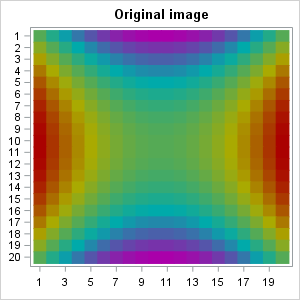The approximation could be improved by using a more sophisticated interpolation scheme, such as bivariate linear interpolation.

Feel free to download the SAS/IML program and play with other weaves. How well can you recover an original image from its weave?
tags: Just for Fun

The post Mathematical art (part 2): Unweaving matrices appeared first on The DO Loop.An artist friend of mine recently created a beautiful abstract image and described the process on her blog. She says that "after painting my initial square, I cut it into strips and split them down the middle, then wove them together.... I had no idea when I started piecing these together again what they would look like."

I marveled at the beauty of the finished result, but I also started thinking about the process of constructing the artwork. You cut an initial picture into n vertical strips. (For simplicity, assume that n is even.) Then you take the first n/2 strips and space them out so that there are gaps between them. You take the last n/2 strips and turn them sideways, weaving them in and out of the vertical strips.

As my friend said in a private communication, the final image "depends entirely on the arrangement of the elements.... There are so many versions of the final outcome." By this she means that the process is deterministic, although the artist can make choices regarding the number of strips, the orientation of the strips, and the weaving pattern. Yes, it is difficult to predict the final image while staring at the initial image, but this is the sort of algorithm that you could ask a computer to carry out.

So, of course, I had to learn to make images like this with SAS!

### Weaving matrices

To make sure that I understood the algorithm, I started with a 10 x 10 numeric matrix X that contains the values 1–100 in row-major order. That is, the first row contains 1–10, the second row contains 11–20, and so forth. I then created a 10 x 10 matrix W (for "weave") that contained all missing values. I copied the first five columns of X into the even columns of W. I then copied the last five columns of X into the even rows of W. I then went back and "wove" the columns and rows by re-copying certain elements from the first five columns of X. You can view this construction process for an example matrix.

The process can be encapsulated into a SAS/IML function, which is shown below:

```proc iml; start weave(x); n = nrow(x); /* n must be even */ y = j(n, n, .); j = 1:n/2; /* vertical strips */ y[, 2*j] = x[, j]; /* copy to every other column */ i = (n/2+1):n; /* horizontal strips */ y[2*i-n,] = x[, i]`; /* copy to every other row */ k = do(1, n/2, 2); /* weaving: raise certain rows */ y[2*k, 2*k] = x[2*k, k]; /* every other for even rows */ k = do(2, n/2, 2); y[2*k, 2*k] = x[2*k,k]; /* every other for odd rows */ return( y ); finish;   x = shape(1:100, 10); /* original 10x10 matrix */ w = weave(x); print w;```

### Weaving heat maps

Now the fun can begin. Recall that a heat map is essentially a visualization of a matrix of numbers. A heat map can be used to visualize mathematical functions of two variables, and you can use the HEATMAPCONT function in SAS/IML 13.1 to easily create a heat map.

You can use the EXPANDGRID function to define a grid of evenly spaced points in the square [-1, 1] x [-1, 1]. You can evaluate a mathematical function on the grid and reshape the results into a matrix. You can then create a heat map of the original function and the "weave" of the function. For details, you can download the complete SAS/IML program that I used to construct the images in this article.

For example, in the following image, the function on the left is f(x,y) = x. The heat map that visualizes the function shows that the function is constant on vertical strips. You can see that each strip is a constant color, and the image on the right has taken those strips and woven them together.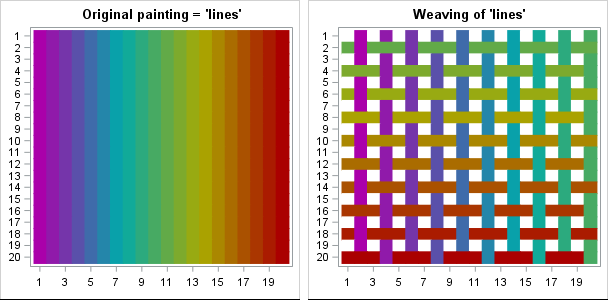The woven image is beautiful, but you can make more interesting images by choosing more interesting functions to use as the original image. The left portion of the following image shows a heat map for the quadratic function f(x,y) = x2 + y2. The image on the right is formed by weaving the vertical strips from the left image.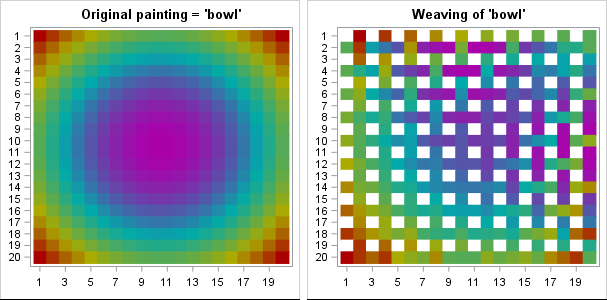You get the idea: You can visualize any mathematical function and then form "mathematical art" by cutting the function along strips and weaving the strips back together.

If you are adventurous, you can play with different color palettes for the images. I chose a muted rainbow palette, but you can experiment with other color ramps. The program for these examples include other color ramps that change the aesthetics of the images.I'll leave you with one more image. It is the weave for a common function from multivariable calculus. Can you deduce the original image from the weave? If not, look in the program that creates these examples..

These images show flexibility of the SAS/IML language for creating a custom algorithm. These simple images lack the depth and complexity of "real" art, but they show how matrices can be used to illustrate the main ideas behind a simple artistic process.

Try it yourself! What images can you make? If you create an interesting picture, leave a comment that includes the mathematical formula.

tags: Just for Fun

The post Mathematical art: Weaving matrices appeared first on The DO Loop.Last week I was chatting with some mathematicians and I mentioned the blog post that I wrote last year on the distribution of Pythagorean triples.

In my previous article, I showed that there is an algorithm that uses matrix multiplication to generate every primitive Pythagorean triple by starting with the simple (3,4,5) right triangle. The algorithm does not systematically generate triangles with large legs from triangles with smaller legs. Instead, it might generate several triangles with large legs, followed by one or more triangles with small legs. Given a specific radius r, it would be interesting to prove how many iterations of the algorithm are required to guarantee that the algorithm has generated all primitive Pythagorean triples whose hypothesis is less than r.

From a statistical point of view, it is interesting to estimate the distribution of the smallest angle in primitive Pythagorean right triangles. Suppose that you consider only the primitive triangles whose hypotenuse is less than 106. The following histogram shows the distribution of the smallest angle (arctan(b/a) if a is the longer leg) for angles ranging from 0 to 45 degrees in increments of 0.5 degrees. The histogram is formed from 98,151 triangles, which is considerably less than the 159,139 primitive right triangles whose hypotenuses are less than one million.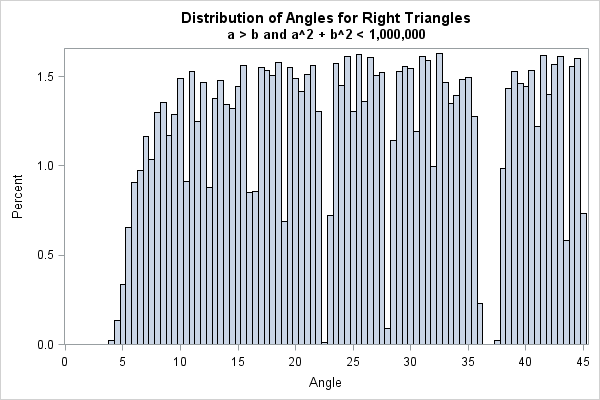The histogram has several interesting features:

• Apparently there are relatively few long-and-skinny triangles. In this sample, few have angles less than 5 degrees. The smallest angle is 3.47 degrees, which corresponds to the (33, 544, 545) right triangle.
• The distribution is not uniform. In fact, there are noticeable gaps in the density distribution. The biggest gaps are near 37, 23, and 28 degrees.
• Some Pythagorean triangles that are nearly isosceles. In this sample, the (137903, 137904, 195025) triangle has an angle equal to 44.99979 degrees. This triangle is an example of a twin-leg Pythagorean triple in which the legs lengths differ by 1.

The gaps are very interesting. I conjecture that the gaps in the distribution are not random, but that they are related to low-order triangles. The following statements compute the smallest angle of some right triangles whose legs are small integers:

```proc iml; v = { 4 3 5, 12 5 13, 15 8 17, 24 7 25, 21 20 29, 35 12 37 }; a = atan2(v[,2], v[,1]) * 180/constant('pi'); print v[L="" c={"a" "b" "c"}] a[L="Angle"];```If you overlay lines at each of the angles above, they fall right into the gaps of the histogram. The (3,4,5) triangle is centered at the biggest gap (37 degrees). The (5, 12, 13) triangle is responsible for the next largest gap (23 degrees), and so forth. You can overlay these lines on the histogram, or overlay the lines on a fringe plot, as shown in the following image in which the red lines represent the angles of six low-order triangles: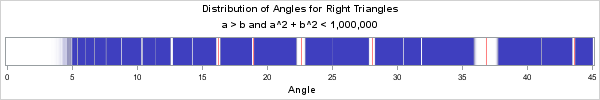If this histogram is representative of the true density distribution of the smallest angle in a Pythagorean triangle, the low-order triangles seem to be surrounded by a region of reduced density. If I were to study this problem further, I would conjecture that the gap induced by a low-order triangle is related to Farey sequences. Farey sequences arise in the darndest places, ranging from the dynamics of planetary objects to the study of Diophantine equations. In fact, the fringe plot reminds me of the gaps and divisions in Saturn's rings, which is another place where Farey sequences have an application. Of course, these gaps could also be an artifact of the algorithm that I am using to generate the primitive Pythagorean triangles, but that can be checked by using a different algorithm to generate the Pythagorean triples. Personally, I think the gaps are produced by a real number-theoretic phenomenon.

The density distribution of the smallest angle is interesting, don't you think? Mathematicians who work in number theory can probably explain why the gaps exist, but they were a surprise to me. Who would have guessed that Pythagorean triangles would lead to such an interesting distribution? It's a beautiful connection between probability, statistics, and high-school geometry.

tags: Just for Fun, Math

The post The distribution of Pythagorean triples by angle appeared first on The DO Loop.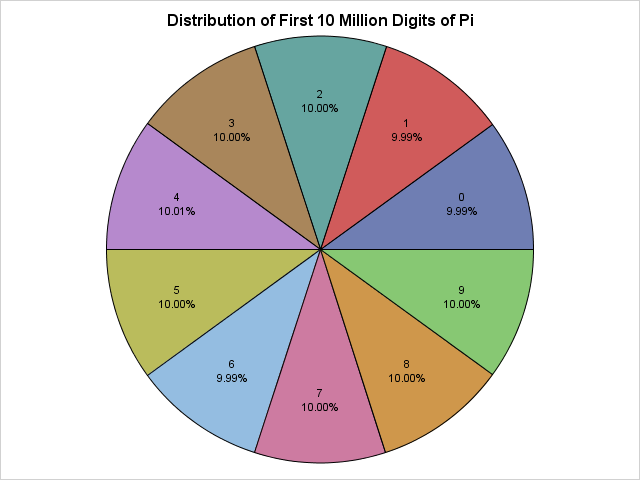Saturday, March 14, 2015, is Pi Day, and this year is a super-special Pi Day! This is your once-in-a-lifetime chance to celebrate the first 10 digits of pi (π) by doing something special on 3/14/15 at 9:26:53. Apologies to my European friends, but Pi Day requires that you represent dates with the month placed first in order to match the sequence 3.141592653....

Last year I celebrated Pi Day by using SAS to explore properties of the continued fraction expansion of pi. This year, I will examine statistical properties of the first 10 million digits of pi. In particular, I will show that the digits of pi exhibit statistical properties that are inherent in a random sequence of integers.

### Reading 10 million digits of pi

I have no desire to type in 10 million digits, so I will use SAS to read a text file at a Princeton University URL. The following statements use the FILENAME statement to point to the URL:

```/* read data over the internet from a URL */ filename rawurl url "http://www.cs.princeton.edu/introcs/data/pi-10million.txt" /* proxy='http://yourproxy.company.com:80' */ ;   data PiDigits; infile rawurl lrecl=10000000; input Digit 1. @@; Position = _n_; Diff = dif(digit); /* compute difference between adjacent digits */ run;   proc print data=PiDigits(obs=9); var Digit; run;```The PiDigits data set contains 10 million rows. The call to PROC PRINT displays the first few decimal digits, which are (skipping the 3) 141592653....

For other ways to use SAS to download data from the internet, search Chris Hemedinger's blog, The SAS Dummy for "PROC HTTP" and you will find several examples of how to download data from a URL.

### The distribution of digits of pi

You can run many statistical tests on these numbers. It is conjectured that the digits of pi are randomly uniformly distributed in the sense that the digits 0 through 9 appear equally often, as do pairs of digits, trios of digits, and so forth.

You can call PROC FREQ to compute the frequency distribution of the first 10 million digits of pi and to test whether the digits appear to be uniformly distributed:

```/* Are the digits 0-9 equally distributed? */ proc freq data = PiDigits; tables Digit/ chisq out=DigitFreq; run;```The frequency analysis of the first 10 million digits shows that each digit appears about one million times. A chi-square test indicates that the digits appear to be uniformly distributed. If you turn on ODS graphics, PROC FREQ also produces a deviation plot that shows that the deviations from uniformity are tiny.

### A "pi chart" of the distribution of the digits of pi

As an advocate of the #OneLessPie Chart Initiative, I am intellectually opposed to creating pie charts. However, I am going to grit my teeth and make an exception for this super-special Pi Day. You can use the Graph Template Language (GTL) to create a pie chart. Even simpler, Sanjay Matange has written a SAS macro that creates a pie chart with minimal effort. The following DATA step create a percentage variable and then calls Sanjay's macro:

```data DigitFreq; set DigitFreq; Pct = Percent/100; format Pct PERCENT8.2; run;   /* macro from http://blogs.sas.com/content/graphicallyspeaking/2012/08/26/how-about-some-pie/ */ %GTLPieChartMacro(data=DigitFreq, category=Digit, response=Pct, title=Distribution of First 10 Million Digits of Pi, DataSkin=NONE);```

The pie chart appears at the top of this article. It shows that the digits 0 through 9 are equally distributed.

### Any autocorrelation in the sequence?

In the DATA step that read the digits of pi, I calculated the difference between adjacent digits. You can use the SGPLOT procedure to create a histogram that shows the distribution of this quantity:

```proc sgplot data=PiDigits(obs=1000000); vbar Diff; run;```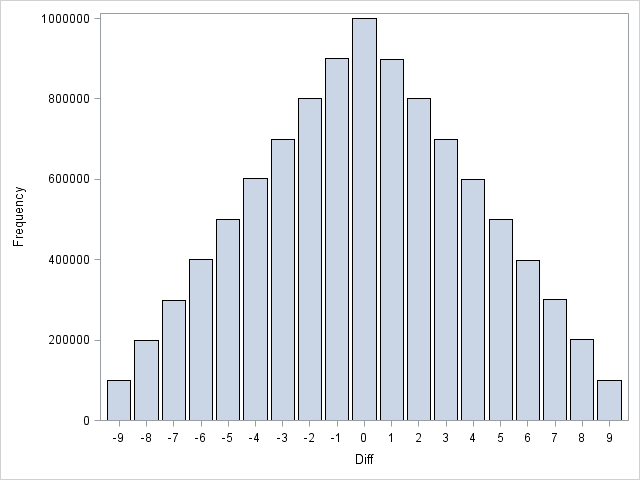That's a pretty cool triangular distribution! I won't bore you with mathematical details, but this shape arises when you examine the difference between two independent discrete uniform random variables, which suggests that the even digits of pi are independent of the odd digits of pi.

In fact, more is true. You can run a formal test to check for autocorrelation in the sequence of numbers. The Durbin-Watson statistic, which is available in PROC REG and PROC AUTOREG, has a value near 2 if a series of values has no autocorrelation. The following call to PROC AUTOREG requests the Durbin-Watson statistic for first-order through fifth-order autocorrelation for the first one million digits of pi. The results show that there is no detectable autocorrelation through fifth order. To the Durban-Watson test, the digits of pi are indistinguishable from a random sequence:

```proc autoreg data=PiDigits(obs=1000000); model Digit = / dw=5 dwprob; run;```### Are the digits of pi random?

Researchers have run dozens of statistical tests for randomness on the digits of pi. They all reach the same conclusion. Statistically speaking, the digits of pi seems to be the realization of a process that spits out digits uniformly at random.

Nevertheless, mathematicians have not yet been able to prove that the digits of pi are random. One of the leading researchers in the quest commented that if they are random then you can find in the sequence (appropriately converted into letters) the "entire works of Shakespeare" or any other message that you can imagine (Bailey and Borwein, 2013). For example, if I assign numeric values to the letters of "Pi Day" (P=16, I=9, D=4, A=1, Y=25), then the sequence "1694125" should appear somewhere in the decimal expansion of pi. I wrote a SAS program to search the decimal expansion of pi for the seven-digit "Pi Day" sequence. Here's what I found:

```proc print noobs data=PiDigits(firstobs=4686485 obs=4686491); var Position Digit; run;```There it is! The numeric representation of "Pi Day" appears near the 4.7 millionth decimal place of pi. Other "messages" might not appear in the first 10 million digits, but this one did. Finding Shakespearian sonnets and plays will probably require computing more digits of pi than the current world record.

The digits of pi pass every test for randomness, yet pi is a precise mathematical value that describes the relationship between the circumference of a circle and its diameter. This dichotomy between "very random" and "very structured" is fascinating! Happy Pi Day to everyone!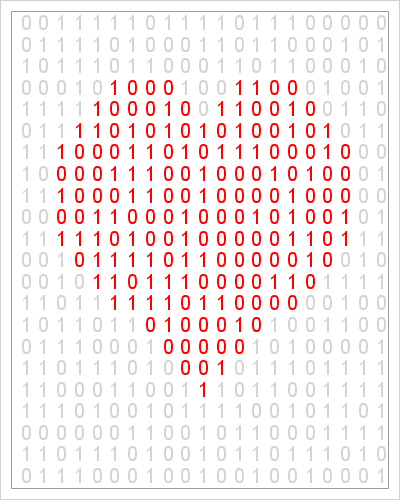The xkcd comic often makes me think and laugh. The comic features physics, math, and statistics among its topics. Many years ago, the comic showed a "binary heart": a grid of binary (0/1) numbers with the certain numbers colored red so that they formed a heart.

Some years later, I used an equation in polar coordinates to draw a heart in SAS as a Valentine's Day gift to my wife. This year I thought it would be fun to combine these two ideas into a geeky Valentine's Day post.

The following SAS DATA step generates on a uniform grid of points near the origin. You can create an indicator variable that specifies which elements of the grid are inside the heart equation. By using the RAND function, you can generate a random 0 or 1 according to the Bernoulli distribution. You can then call the SGPLOT procedure to show the binary values, using red to display those that are inside the heart region and light gray to display the values outside the heart. The resulting image is shown at the top of this article.

```/* generate random binary heart similar to xkcd comic: http://xkcd.com/99/ */ data BinaryHeart; drop Nx Ny t r; Nx = 21; Ny = 23; call streaminit(2142015); do x = -2.6 to 2.6 by 5.2/(Nx-1); do y = -4.4 to 1.5 by 6/(Ny-1); /* convert (x,y) to polar coordinates (r, t) */ r = sqrt( x**2 + y**2 ); t = atan2(y,x); /* eqn: blogs.sas.com/content/iml/2011/02/14/a-parametric-view-of-love/ */ Heart= (r < 2 - 2*sin(t) + sin(t)*sqrt(abs(cos(t))) / (sin(t)+1.4)) & (y > -3.5); B = rand("Bernoulli", 0.5); output; end; end; run;   ods graphics / width=400px height=500px; title "Happy Valentine's Day"; proc sgplot data=BinaryHeart noautolegend; styleattrs datacontrastcolors=(lightgray red); scatter x=x y=y / group=Heart markerchar=B markercharattrs=(size=14); xaxis display=none offsetmin=0 offsetmax=0.06; yaxis display=none; run;```

The image is similar to the original hand-drawn xkcd comic, although the random binary values are different. I don't know what a "binary heart" means, but I find it interesting nonetheless.

Notice the use of the STYLEATTRS statement in PROC SGPLOT to set the colors of the marker characters. The STYLEATTRS statement was added in SAS 9.4. In SAS 9.3 you can use the DATTRMAP= option in the PROC SGPLOT statement to specify the colors of groups in SAS statistical graphics.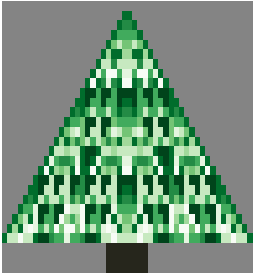O Christmas tree,
O Christmas tree,
One year a fractal made thee!
O Christmas tree,
O Christmas tree,
A heat map can display thee!

From Pascal's matrix we define!
Reflect across, divide by nine.
O Christmas tree,
O Christmas tree,
Self-similar and so divine!

Eventually I will run out of cute ways to use SAS software to create and visualize a Christmas-themed image. But not this year!

My recent article about how to create Pascal's matrix in SAS included a lower triangular matrix that exhibits self-similar patterns. If you take the Pascal matrix modulo 9 and reflect it across the Y axis, you get a triangular array that looks a little bit like a Christmas tree. You can add a "trunk" to the bottom of the tree to improve the resemblance. As shown in my previous post, you can use a heat map to visualize the matrix. In the following SAS/IML program, I use a green palette of colors to visualize the Pascal triangle values modulo 9. The resulting heat map is shown at the top of this article.

```proc iml; start PascalRule(n); m = j(n,n,0); /* initialize with zeros */ m[,1] = 1; /* set first column to 1 */ j = 2:n; /* elements to compute */ do k = 2 to n; /* for kth row, add adjacent elements from previous row */ m[k,j] = m[k-1,j-1] + m[k-1,j]; end; return(m); finish;   /* reflect Pascal's triangle to create left-right symmetry and add a tree trunk to create a Christmas tree image */ start PascalTree(n); m = PascalRule(n); T = m[,n:2] || m[,2:n]; /* reflect; omit column of 1s */ T[ loc(T=0) ] = .; /* replace zeros with missing values */ trunk = j(3,ncol(T),.); /* add a "tree trunk" with value -1 */ midpt = ncol(T)/2; /* note that ncol(T) is even */ halfwidth = ceil(n/10); trunk[,(midpt-halfwidth):(midpt+halfwidth+1)]= -1; return( T // trunk ); finish;   m = PascalTree(25); k = 9; tree = mod(m,k);   ods graphics / width=300px height=380px; title = "Happy Holidays to All SAS Users!"; ramp = palette("greens", k); ramp = "CX26261C" || ramp[,k:1]; /* brown (for trunk) and green palette */ call heatmapdisc(tree) colorramp=ramp displayoutlines=0 title=title;```

It is remarkable that the Pascal matrix has so many amazing mathematical properties. Now you can add "makes a reasonable facsimile of a Christmas tree" to the list!

Happy holidays and a wonderful New Year to all of my readers. You are the reason that I write this blog.

tags: Heat maps, Just for FunPascal's triangle is the name given to the triangular array of binomial coefficients. The nth row is the set of coefficients in the expansion of the binomial expression (1 + x)n. Complicated stuff, right?

Well, yes and no. Pascal's triangle is known to many school children who have never heard of polynomials or coefficients because there is a fun way to construct it by using simple addition. You start by writing down the number 1. The second line is the sum of the first line and the result of shifting the first line one column to the right. This process continues: to form a new row of the triangle, add the previous row to a right-shifted copy of the previous row. To make the bookkeeping work out, you pretend that missing items are zero, as shown by the ghostly images of zeros in the image at the top of this article.

In math terms, you can find the kth element of the nth row, by adding the (k–1)th and the kth elements of the previous row. This geometric construction is a result of the following recursive relationship between binomial coefficients: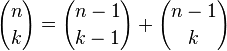Recently several participants on the SAS/IML Support Community posted programs that created Pascal's triangle by using the COMB function in SAS software. This article shows how to create Pascal's triangle and how self-similar (fractal) patterns arise when you visualize the triangle in certain ways.

### Creating an array of binomial coefficients

The simplest way to create Pascal's matrix is to use the COMB function to generate the values of the binomial coefficients. You can start with a matrix that contains all zeros. The first k elements of the kth row are filled with binomial coefficients. You can do this in the SAS DATA step, or in the SAS/IML language as follows:

```proc iml; start PascalTriangle(n); m = j(n,n,0); /* matrix with all zeros */ do k = 1 to n; /* for the kth row... */ m[k,1:k] = comb(k-1, 0:k-1); /* fill nonzero elements */ end; return(m); finish;   T10 = PascalTriangle(10); print T10[F=3. L="Pascal's Triangle, Level 10" r=("n=0":"n=9")];```

The resulting matrix is similar to the image at the top of this post, with the upper triangular elements equal to zero. Notice that the SAS/IML language enables you to pass in a vector argument to a Base SAS function. In this case, a vector (0:k-1) is passed to the COMB function and the resulting row vector overwrites some of the zeros in the matrix m.

### Using the previous row to create the next row of Pascal's matrix

You can also create Pascal's matrix by using the "schoolchild method" of adding adjacent elements from the previous row to create the new row. The construction is similar to the way that you can construct cellular automata. The following SAS/IML module constructs Pascal's triangle by using addition; no need to call the COMB function!

```start PascalRule(n); m = j(n,n,0); /* initialize with zeros */ m[,1] = 1; /* set first column to 1 */ j = 2:n; /* elements to compute */ do k = 2 to n; /* for kth row, add adjacent elements from previous row */ m[k,j] = m[k-1,j-1] + m[k-1,j]; end; return(m); finish;   T10 = PascalRule(10); print T10[F=3. L="Pascal's Triangle, Level 10" r=("n=0":"n=9")];```

### Self-similar structures in Pascal's triangle

At first glance, the numbers in Pascal triangle have a simple structure. The edges of the triangle are all 1. The interior values increase geometrically, reaching their maximum values in the middle of the final row. However, if you label each value according to whether it is odd or even, a surprising pattern reveals itself!

The following SAS/IML program creates a Pascal matrix with 56 rows. The upper-triangular elements are set to missing values. The program then creates a discrete heat map that shows the parity (even or odd) of the remaining elements. Even numbers are displayed as white squares; odd numbers are displayed as black squares.
```ods graphics / width=400px height=380px; m = PascalRule(56); m[ loc(col(m)>row(m)) ] = .; /* replace zeros with missing values */ mod2 = mod(m,2); call heatmapdisc(mod2) colorramp={WHITE BLACK} displayoutlines=0 title="Pascal's Triangle mod 2";```The resulting heat map bears a striking resemblance to the fractal known as Sierpinski's triangle. This fact is not widely known, but the image is comprehensible to school-age children. However, few children have the patience and stamina to color hundreds of cells, so using software to color the triangle is definitely recommended!

The fascinating self-similar pattern might inspire you to wonder what happens if the elements are colored according to some other scheme. For example, what is the pattern if you divide the elements of Pascal's triangle by 3 and visualize the remainders? Or division by 4? Or 5? The following loop creates three heat maps that visualize the numbers in Pascal's triangle modulo k, where k = 3, 4, and 5.

```do k = 3 to 5; mod = mod(m,k); ramp = palette("greys", k); title = "Pascal's Triangle mod " + char(k,1); call heatmapdisc(mod) colorramp=ramp displayoutlines=0 title=title; end;```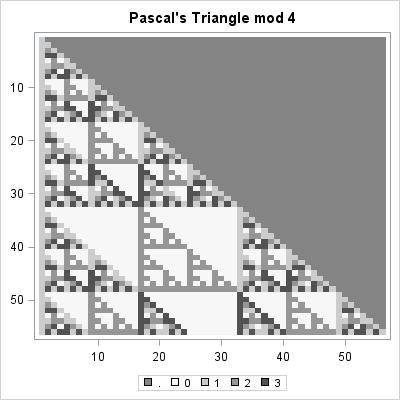The "mod 4" result is shown. The other heat maps are similar. Each shows a self-similar pattern. One of the surprising results of chaos theory and fractals is that these complicated self-similar structures can arise from simple iterative arithmetic operations (adding adjacent elements) followed by a modular operation.

### Creating larger triangles

The astute reader will have noticed that 56 rows is a curious number. Why not 64 rows? Or 100? The answer is that the modular operations require that the numbers in Pascal's triangle be exactly representable as an integer. Although you can compute more than 1,000 rows of Pascal's triangle in double precision, at some point the numbers grow so large that they can no longer be represented exactly with an 8-byte integer.

You can use the SAS CONSTANT function to find the largest integer, B, such that smaller integers (in magnitude) are exactly represented by an 8-byte numeric value. It turns out that the largest value in a Pascal triangle with k rows is "k choose floor(k/2)," and this value exceeds B when k=57, as shown by the following statements. Thus the modulo operations will become inaccurate for k>56.

```B = constant('ExactInt'); print B;   k = T(55:58); c = comb(k, floor(k/2)); print k c[L="k choose [k/2]"] (c<B)[L="Exact?"];```I think Pascal's triangle is very cool. When did you first encountered Pascal's triangle? Were you fascinated or bored? Share your story in the comments.

tags: Heat maps, Just for Fun, MathWhen I studied high school geometry, I noticed that many homework problems involved right triangles whose side lengths were integers. The canonical example is the 3-4-5 right triangle, which has legs of length 3 and 4 and a hypotenuse of length 5. The triple (3, 4, 5) is called a Pythagorean triple because it satisfies the Pythagorean theorem: 32 + 42 = 52. Similarly, (5, 12, 13) and (7, 24, 25) are Pythagorean triples that sometimes appear in geometry textbooks.

In general, a triple of natural numbers (a, b, c) is a Pythagorean triple if a2 + b2 = c2. Obviously if (a, b, c) is a Pythagorean triple, then so is (ka, kb, kc) for any natural number k. Geometrically, this transformation is not very interesting because it simply scales a right triangle into a similar triangle that has the same shape. I did not learn any trick in high school that enabled me to start with a Pythagorean triple and generate a new, geometrically different, right triangle.

### Generating Pythagorean triples

Because my high school geometry book reused the same three or four triples over and over again, I assumed that it is difficult to generate Pythagorean triples. In fact, that is not the case: There are several formulas for generating Pythagorean triples, some of which were known to the ancient Greeks.

One interesting algorithm comes from the 20th-century and involves linear transformations via matrices. Here's how it works. Write any Pythagorean triple, v, as a row vector. Then the linear transformation v*L is a new Pythagorean triple, where L is any of the three following linear transformations: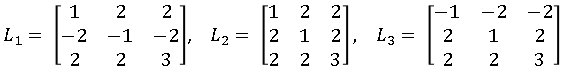For example, if you represent v = (3, 4, 5) as a row vector, then v*L1 = (5, 12, 13). You can multiply on the right again to get another new triple, such as v*L1*L3 = (45, 28, 53).

In fact, more is true. A primitive Pythagorean triple is a triple such that the three numbers have no nontrivial divisors, that is, they are relatively prime. It turns out that all primitive Pythagorean triples can be obtained by iteratively applying one of three linear transformations to the triple (3, 4, 5). In other words, the triple (3, 4, 5) is the "parent" of all primitive Pythagorean triples!

That is a fabulous result. It means that you can write a program that uses matrix multiplication to produce arbitrarily many primitive Pythagorean triples, as follows:

• Apply L1, L2, and L3 to create the first generation of children.
• Apply the three transformations to each of the children to create a generation of nine grandchildren.
• Continue this process. The ith generation will contain 3i triples.

You can implement this algorithm in the SAS/IML matrix language as follows:

```proc iml; start GenerateTriples(depth=3); L1 = { 1 2 2, -2 -1 -2, 2 2 3}; L2 = { 1 2 2, 2 1 2, 2 2 3}; L3 = {-1 -2 -2, 2 1 2, 2 2 3}; NumTriples = sum(3##(0:Depth)); /* total number of triples */ triples = j(NumTriples,3,.); /* allocate array for results */ triples[1,] = {3 4 5}; /* parent triple */ k = 1; n = 2; do i = 1 to Depth; /* for each generation */ do j = 1 to 3##(i-1); /* generate 3##i children */ x = triples[k,]; triples[n,] = x*L1; triples[n+1,] = x*L2; triples[n+2,] = x*L3; k = k + 1; n = n + 3; end; end; return( triples ); finish;   p = GenerateTriples(); print p;```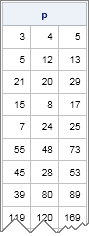The parent and the first three generations account for 1 + 3 + 9 + 27 = 40 triples. The list contains some old familiar friends, as well as a few strangers that I haven't met before.

### The distribution of Pythagorean triples

What do the Pythagorean triples look like if you plot their location in a Cartesian coordinate system? It turns out that they make fascinating patterns!

Because the hypotenuse length is a function of the leg lengths, it suffices to plot the locations of a and b in the coordinate plane. However, it turns out that when the algorithm produces a particular triple such as (5, 12, 13), it does not produce the symmetric triple (12, 5, 13). To make the pattern more symmetric, we can to manually add the triple (b, a, c) whenever the triple (a, b, c) appears.

The following SAS/IML statements construct many primitive Pythagorean triples and create a scatter plot of the ones for which a and b are both less than 4,500:

```p = GenerateTriples(15); /* generate many triples */ m = p[ loc(p[,1]<=4500 & p[,2]<=4500), ]; /* exclude large ones */ mm = m // m[,{2 1 3}]; /* generate symmetric pairs */   ods graphics / width=600px height=600px; title "Primitive Pythagorean Triples"; title2 "Depth = 15, Symmetric"; call scatter(mm[,1], mm[,2]) procopt="aspect=1" option="markerattrs=(size=3 symbol=SquareFilled)";```

The image is shown at the beginning of this article. You can see certain parabolic curves that have a high density of points. There is a similar plot in Wikipedia that includes nonprimitive triples. The nonprimitive triples "fill in" some of the apparent "gaps" in the pattern.

These interesting patterns hint at deep relationships in number theory between Diophantine equations and the geometry of the solutions. Are you tempted to generate a similar image for the generalized equation a3 + b3 = c3? Don't bother: Fermat's Last Theorem states that this equation has no integer solutions!

tags: Just for Fun, MathWhen I studied high school geometry, I noticed that many homework problems involved right triangles whose side lengths were integers. The canonical example is the 3-4-5 right triangle, which has legs of length 3 and 4 and a hypotenuse of length 5. The triple (3, 4, 5) is called a Pythagorean triple because it satisfies the Pythagorean theorem: 32 + 42 = 52. Similarly, (5, 12, 13) and (7, 24, 25) are Pythagorean triples that sometimes appear in geometry textbooks.

In general, a triple of natural numbers (a, b, c) is a Pythagorean triple if a2 + b2 = c2. Obviously if (a, b, c) is a Pythagorean triple, then so is (ka, kb, kc) for any natural number k. Geometrically, this transformation is not very interesting because it simply scales a right triangle into a similar triangle that has the same shape. I did not learn any trick in high school that enabled me to start with a Pythagorean triple and generate a new, geometrically different, right triangle.

### Generating Pythagorean triples

Because my high school geometry book reused the same three or four triples over and over again, I assumed that it is difficult to generate Pythagorean triples. In fact, that is not the case: There are several formulas for generating Pythagorean triples, some of which were known to the ancient Greeks.

One interesting algorithm comes from the 20th-century and involves linear transformations via matrices. Here's how it works. Write any Pythagorean triple, v, as a row vector. Then the linear transformation v*L is a new Pythagorean triple, where L is any of the three following linear transformations:For example, if you represent v = (3, 4, 5) as a row vector, then v*L1 = (5, 12, 13). You can multiply on the right again to get another new triple, such as v*L1*L3 = (45, 28, 53).

In fact, more is true. A primitive Pythagorean triple is a triple such that the three numbers have no nontrivial divisors, that is, they are relatively prime. It turns out that all primitive Pythagorean triples can be obtained by iteratively applying one of three linear transformations to the triple (3, 4, 5). In other words, the triple (3, 4, 5) is the "parent" of all primitive Pythagorean triples!

That is a fabulous result. It means that you can write a program that uses matrix multiplication to produce arbitrarily many primitive Pythagorean triples, as follows:

• Apply L1, L2, and L3 to create the first generation of children.
• Apply the three transformations to each of the children to create a generation of nine grandchildren.
• Continue this process. The ith generation will contain 3i triples.

You can implement this algorithm in the SAS/IML matrix language as follows:

```proc iml; start GenerateTriples(depth=3); L1 = { 1 2 2, -2 -1 -2, 2 2 3}; L2 = { 1 2 2, 2 1 2, 2 2 3}; L3 = {-1 -2 -2, 2 1 2, 2 2 3}; NumTriples = sum(3##(0:Depth)); /* total number of triples */ triples = j(NumTriples,3,.); /* allocate array for results */ triples[1,] = {3 4 5}; /* parent triple */ k = 1; n = 2; do i = 1 to Depth; /* for each generation */ do j = 1 to 3##(i-1); /* generate 3##i children */ x = triples[k,]; triples[n,] = x*L1; triples[n+1,] = x*L2; triples[n+2,] = x*L3; k = k + 1; n = n + 3; end; end; return( triples ); finish;   p = GenerateTriples(); print p;```The parent and the first three generations account for 1 + 3 + 9 + 27 = 40 triples. The list contains some old familiar friends, as well as a few strangers that I haven't met before.

### The distribution of Pythagorean triples

What do the Pythagorean triples look like if you plot their location in a Cartesian coordinate system? It turns out that they make fascinating patterns!

Because the hypotenuse length is a function of the leg lengths, it suffices to plot the locations of a and b in the coordinate plane. However, it turns out that when the algorithm produces a particular triple such as (5, 12, 13), it does not produce the symmetric triple (12, 5, 13). To make the pattern more symmetric, we can to manually add the triple (b, a, c) whenever the triple (a, b, c) appears.

The following SAS/IML statements construct many primitive Pythagorean triples and create a scatter plot of the ones for which a and b are both less than 4,500:

```p = GenerateTriples(15); /* generate many triples */ m = p[ loc(p[,1]<=4500 & p[,2]<=4500), ]; /* exclude large ones */ mm = m // m[,{2 1 3}]; /* generate symmetric pairs */   ods graphics / width=600px height=600px; title "Primitive Pythagorean Triples"; title2 "Depth = 15, Symmetric"; call scatter(mm[,1], mm[,2]) procopt="aspect=1" option="markerattrs=(size=3 symbol=SquareFilled)";```

The image is shown at the beginning of this article. You can see certain parabolic curves that have a high density of points. There is a similar plot in Wikipedia that includes nonprimitive triples. The nonprimitive triples "fill in" some of the apparent "gaps" in the pattern.

These interesting patterns hint at deep relationships in number theory between Diophantine equations and the geometry of the solutions. Are you tempted to generate a similar image for the generalized equation a3 + b3 = c3? Don't bother: Fermat's Last Theorem states that this equation has no integer solutions!

tags: Just for Fun, Math